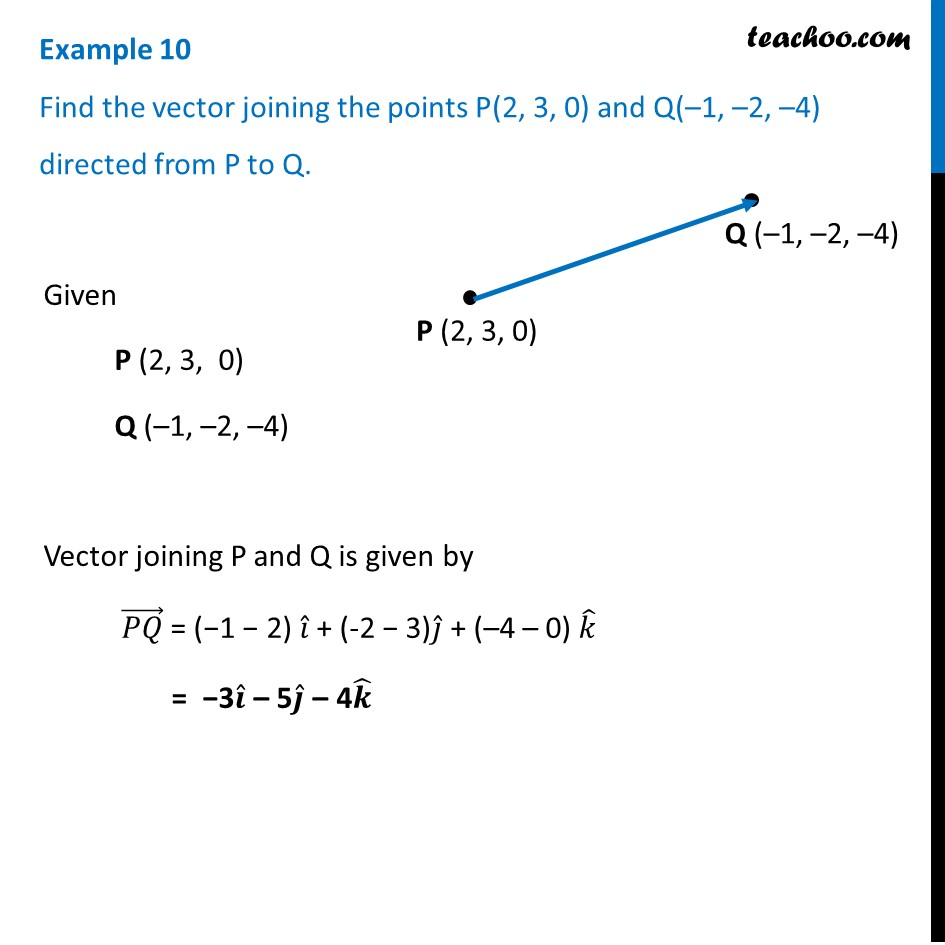Examples

Chapter 10 Class 12 Vector Algebra
Serial order wiseLearn in your speed, with individual attention - Teachoo Maths 1-on-1 Class

### Transcript

Example 10 Find the vector joining the points P(2, 3, 0) and Q(–1, –2, –4) directed from P to Q. Given P (2, 3, 0) Q (–1, –2, –4) Vector joining P and Q is given by (𝑃𝑄) ⃗ = (−1 − 2) 𝑖 ̂ + (-2 − 3)𝑗 ̂ + (–4 – 0) 𝑘 ̂ = −3𝒊 ̂ – 5𝒋 ̂ – 4𝒌 ̂